### 1.6 Numbers

Numbers are by default denoted in decimal notation. Real (or decimal) numbers are written using engineering or scientific notation (e. g. 0.314E1).

For integer type constants, Free Pascal supports four formats:

1. Normal, decimal format (base 10). This is the standard format.
2. Hexadecimal format (base 16), in the same way as Turbo Pascal does. To specify a constant value in hexadecimal format, prepend it with a dollar sign (\$). Thus, the hexadecimal \$FF equals 255 decimal. Note that case is insignificant when using hexadecimal constants.
3. As of version 1.0.7, Octal format (base 8) is also supported. To specify a constant in octal format, prepend it with an ampersand (&). For instance 15 is specified in octal notation as &17.
4. Binary notation (base 2). A binary number can be specified by preceding it with a percent sign (%). Thus, 255 can be specified in binary notation as %11111111.

The following diagrams show the syntax for numbers.

_________________________________________________________________________________________________________
Numbers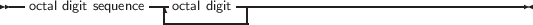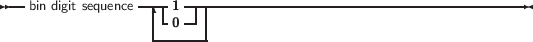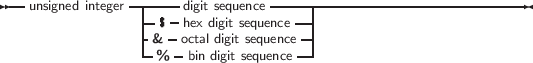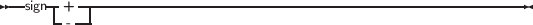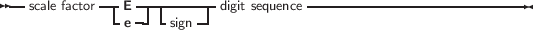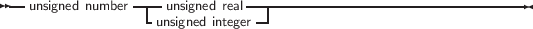___________________________________________________________________

Remark Octal and Binary notation are not supported in TP or Delphi compatibility mode.